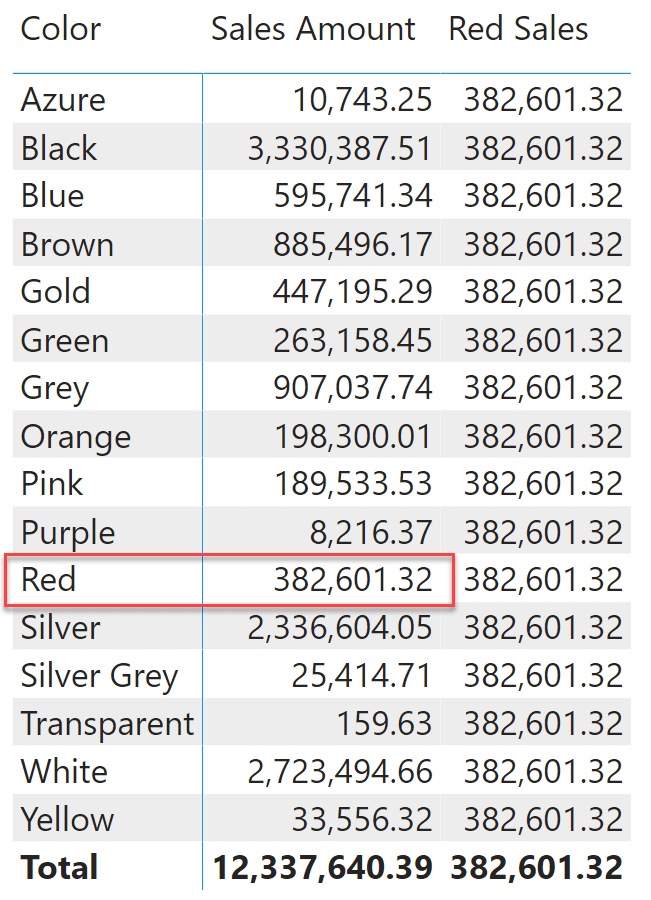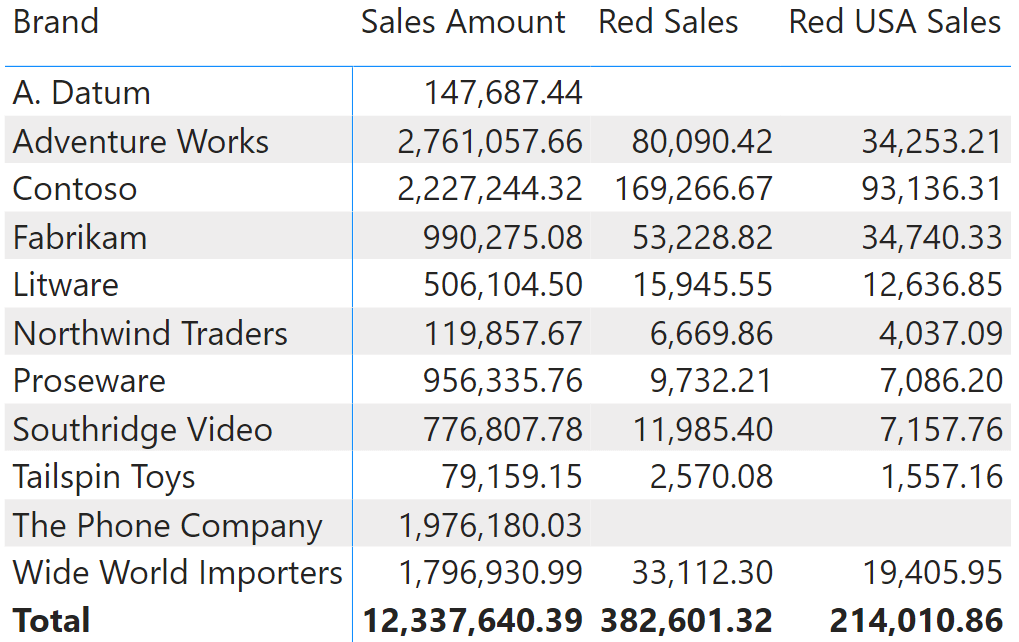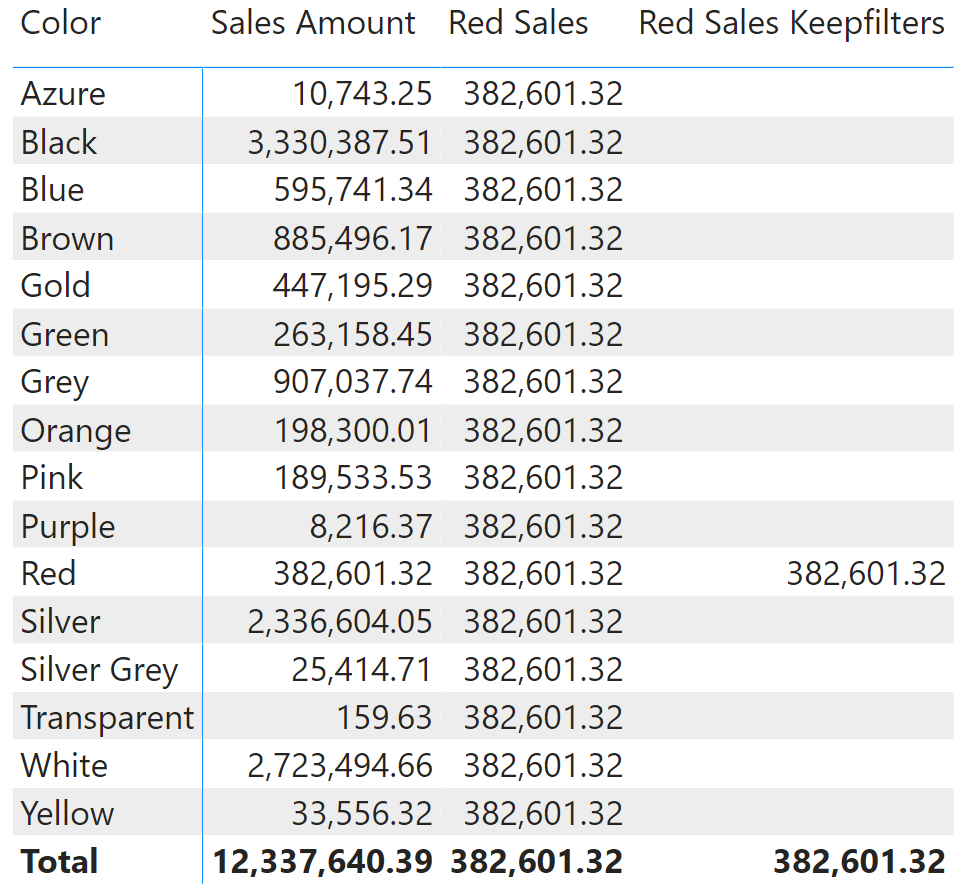CALCULATE, with its companion function CALCULATETABLE, is the only function in DAX that can change the filter context. Its use is very intuitive at first, and most DAX developers start using CALCULATE without knowing the most intricate details of its behavior. Then, sooner than later the use of CALCULATE becomes frightening because CALCULATE starts to misbehave. When this happens, it is nothing but a signal that you need to learn more theory and deepen your understanding of the behavior of CALCULATE.

In this article, we do not introduce the most complex behaviors of CALCULATE. Instead, we provide a beginner’s guide to CALCULATE, and we try to avoid making things simpler than they are. CALCULATE is definitely a complex function. Here we introduce its base behaviors, with a solid theoretical foundation.

Last note before we start: it is impossible to properly understand the details of CALCULATE without a proper understanding of the row context, the filter context and the context transition. If you are not familiar with these concepts, we suggest that you read these articles and gain some practice, then come back to this article.

The syntax of CALCULATE is extremely simple. You invoke CALCULATE with an expression as its first argument, and a set of filters starting from the second parameter onwards. For example, the following measure computes the sales amount of Red products:

Measure in the Sales table
```
Red Sales :=
CALCULATE (
[Sales Amount],
'Product'[Color] = "Red"
)
```

When used in a matrix, the filter over Product[Color] is added to the already-existing filter placed by the matrix itself on the Product[Brand] column.In the first column, when Product[Brand] is in the filter context filtering Contoso, the Sales Amount measure computes the sales amount for Contoso products. When Red Sales is being executed, it calls Sales Amount again, this time adding Product[Color] = “Red” as an additional filter in the filter context.

At this point, let us introduce the first of CALCULATE’s secrets. Even though in the syntax we showed earlier we used a condition to apply the filter over Product[Color], filter arguments of CALCULATE are not conditions: they are tables. Writing a condition like ‘Product'[Color] = “Red” is a compact way to define a table containing the red color. Indeed, even though we use conditions, the DAX engine transforms conditions into tables. The previous code can be written in the following, equivalent way:

Measure in the Sales table
```
Red Sales :=
CALCULATE (
[Sales Amount],
FILTER (
ALL ( 'Product'[Color] ),
'Product'[Color] = "Red"
)
)
```

The syntax using conditions is known as the short syntax, whereas this latter way of expressing the condition is the long syntax. They are not just equivalent. They are the same expression. The short syntax is converted into the long syntax by the engine, before it executes the code. Think of the short syntax as being a convenient way for humans to read the expression. Computers prefer the long syntax, as it is a bit more precise.

The long syntax has the advantage of clarifying the semantics of the filter. FILTER is scanning ALL ( Product[Color] ), therefore it is returning “any product that is red”. This is relevant because CALCULATE can be executed from inside a filter context that is already filtering Product[Color]. In that scenario, the presence of ALL means that the outer filter over Product[Color] is ignored and replaced with the new filter introduced by CALCULATE. This is evident if instead of slicing by Brand, we slice by Color in the matrix.As you see, the value of sales for the red products is repeated in all the rows. For every row, the filter introduced by the matrix is the corresponding color (Black, Blue, Brown…). Red Sales imposes a new filter asking for red to be visible, and this new filter overrides the outer filter so that Sales Amount is computed within a filter context that filters only red products.

This behavior was not entirely evident in the previous figure, because we were slicing by a different column. The filter replacement happens only when the same column is present in both the outer and the inner filter. If the outer filter is filtering a different column, then no replacement takes place. By outer filter we mean the filter context in which CALCULATE is invoked, whereas the inner filter is the one created by CALCULATE after it has modified the outer filter.

You can specify multiple filters in one same CALCULATE. All the filters are intersected together, regardless of the order in which they appear. For example, the following measure computes Sales Amount for red products in the USA:

Measure in the Sales table
```Red USA Sales :=
CALCULATE (
[Sales Amount],
'Product'[Color] = "Red",
Customer[Country] = "United States"
)
```

The result is obviously smaller than Red Sales, as it takes fewer rows in the Sales table into account.As introduced earlier, the new filter introduced by CALCULATE overrides any filters existing previously on the column. You have the option of changing this behavior and making CALCULATE add the new filter onto any previous filter, thus avoiding the override operation. In order to obtain this behavior, you need to use a CALCULATE modifier: KEEPFILTERS.

You can check out the behavior of KEEPFILTERS by authoring a new measure:

Measure in the Sales table
```
Red Sales Keepfilters :=
CALCULATE (
[Sales Amount],
KEEPFILTERS ( 'Product'[Color] = "Red" )
)
```

Red Sales Keepfilters behaves a lot like Red Sales, when the Product[Color] column is not already filtered. Nonetheless, when there is already a filter active on Product[Color], KEEPFILTERS ensures that the outer filter is not overridden, but rather merged with the new filter. You can appreciate the difference between Red Sales and Red Sales Keepfilters in the following matrix.KEEPFILTERS is the first of many CALCULATE modifiers. Other modifiers include USERELATIONSHIP, CROSSFILTER, the entire family of the ALL* functions like ALL, ALLEXCEPT, ALLNOBLANKROW, ALLSELECTED and REMOVEFILTERS – which despite not starting with “ALL” is still part of the ALL* family. There is no room to cover all these functions in this introductory article, but it seemed useful to enumerate the modifiers as a reference.

Many newbies use a different formula to achieve a goal similar to what KEEPFILTERS would do. Instead of using KEEPFILTERS, they use a pattern that includes a filter on the Product table:

Measure in the Sales table
```
Red Sales Table Filter :=
CALCULATE (
[Sales Amount],
FILTER (
'Product',
'Product'[Color] = "Red"
)
)
```

Indeed, Red Sales Table Filter produces the same outcome as Red Sales Keepfilters. Nonetheless, it does so by using a filter over the entire Product table. A table filter is much larger than a single column filter. As such, the expression is going to be slower. Besides, using table filters in CALCULATE results in the filter being applied on the expanded table, which results in even slower queries and potentially semantically incorrect calculations. Expanded tables are an advanced DAX topic that we do not cover in this article. If you are interested in deepening your knowledge on the topic, you can read this article: Expanded tables in DAX.

At this point it is useful to understand exactly how Red Sales Table Filter works. When CALCULATE starts, it follows a very precise order that you can find described on the dax.guide website. Here, we focus on a subset of the entire set of operations.

The first thing that CALCULATE does is to evaluate the filter arguments. This means that the filter arguments of CALCULATE are computed when the outer filter context is still active. Therefore, FILTER iterates the Product table as visible in the outer filter context. If the outer filter context contains a filter for blue products for example, then FILTER iterates over only the blue products. Out of all the blue products, it retrieves the ones that happen to be red. Obviously, there will be none; therefore FILTER returns an empty table that – when later applied as a filter – produces a blank result.

This last detail is important. Filter arguments in CALCULATE are evaluated independently from each other and in the outer filter context. CALCULATE will modify the filter context by applying its filters, but this is the last operation it does. When filter arguments are being evaluated, CALCULATE has not modified the filter context yet.

There is still another operation that CALCULATE performs as part of its job: the context transition. Context transition is a complex topic in itself, therefore we dedicated a full article to the context transition and the correct way to use it. You find more information about the context transition in a specific article.

The complexity of CALCULATE is not in the function itself. CALCULATE only modifies the outer filter context by applying new filters, and it can do so by either overriding the outer filters or combining new filters with the outer filters. The choice is up to the developer, by using KEEPFILTERS or not.

The evaluation contexts and CALCULATE are the foundation of the entire DAX language. This is the reason we created a suite of books, courses, and videos about these important concepts. In this article, we merely scratched the surface. If you want to learn more, then start by watching the free Introducing DAX video course. Once you have digested that content, proceed with one of our in-person classroom courses and/or with watching the Mastering DAX online video course.

CALCULATEContext transition

Evaluates an expression in a context modified by filters.

`CALCULATE ( <Expression> [, <Filter> [, <Filter> [, … ] ] ] )`

CALCULATETABLEContext transition

Evaluates a table expression in a context modified by filters.

`CALCULATETABLE ( <Table> [, <Filter> [, <Filter> [, … ] ] ] )`

FILTERReturns a table that has been filtered.

`FILTER ( <Table>, <FilterExpression> )`

ALLCALCULATE modifier

Returns all the rows in a table, or all the values in a column, ignoring any filters that might have been applied.

`ALL ( [<TableNameOrColumnName>] [, <ColumnName> [, <ColumnName> [, … ] ] ] )`

KEEPFILTERSCALCULATE modifier

Changes the CALCULATE and CALCULATETABLE function filtering semantics.

`KEEPFILTERS ( <Expression> )`

USERELATIONSHIPCALCULATE modifier

Specifies an existing relationship to be used in the evaluation of a DAX expression. The relationship is defined by naming, as arguments, the two columns that serve as endpoints.

`USERELATIONSHIP ( <ColumnName1>, <ColumnName2> )`

CROSSFILTERCALCULATE modifier

Specifies cross filtering direction to be used in the evaluation of a DAX expression. The relationship is defined by naming, as arguments, the two columns that serve as endpoints.

`CROSSFILTER ( <LeftColumnName>, <RightColumnName>, <CrossFilterType> )`

ALLEXCEPTCALCULATE modifier

Returns all the rows in a table except for those rows that are affected by the specified column filters.

`ALLEXCEPT ( <TableName>, <ColumnName> [, <ColumnName> [, … ] ] )`

ALLNOBLANKROWCALCULATE modifier

Returns all the rows except blank row in a table, or all the values in a column, ignoring any filters that might have been applied.

`ALLNOBLANKROW ( <TableNameOrColumnName> [, <ColumnName> [, <ColumnName> [, … ] ] ] )`

ALLSELECTEDCALCULATE modifier

Returns all the rows in a table, or all the values in a column, ignoring any filters that might have been applied inside the query, but keeping filters that come from outside.

`ALLSELECTED ( [<TableNameOrColumnName>] [, <ColumnName> [, <ColumnName> [, … ] ] ] )`

REMOVEFILTERSCALCULATE modifier

Clear filters from the specified tables or columns.

`REMOVEFILTERS ( [<TableNameOrColumnName>] [, <ColumnName> [, <ColumnName> [, … ] ] ] )`